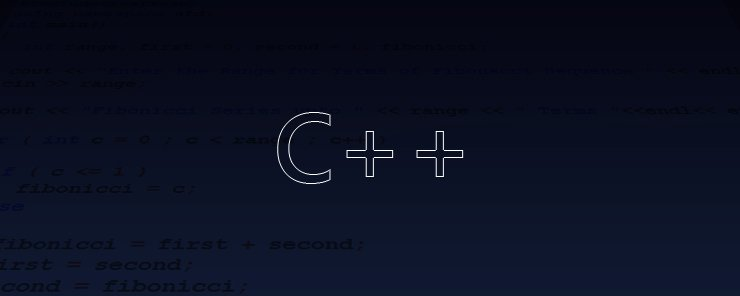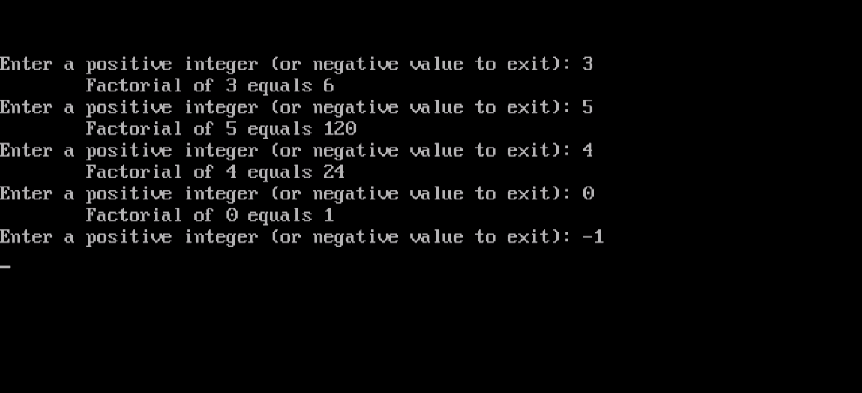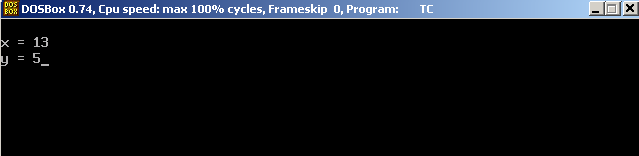# Learn about Functions in C++ Programming

0
2043There will be cases wherein a group of statements is required to be executed in different parts of a program. This can be achieved in one of two ways: the first is to copy and paste the code block wherever it is needed. The second: is to give a name to that block of code, and call it on need. The first approach looks easy, and fast to implement, but at the same time it is very error-prone and difficult to maintain. Any single typo that exists, will have to be fixed in each part the block. Similarly, if the code is to be modified, this has to be done in each part. This is a difficult, boring, and error-prone process. Well, so you may have concluded that I am encouraging you to use the second approach. That is right! This method is called Function, and it is going to be the topic of this article. A very important topic really, so be with us, and you won’t regret it.

Why Functions?
Besides the reason we mentioned in the above introduction (facilitating maintenance and reducing typo errors), using functions can also help keep your program at suitable size.

Function Types
There are two main types of functions: built-in functions, and user-defined functions. The built-in functions are ready-made functions that the C/C++ has created for you to use. We have seen several instances of this type: for example, the main() function, which is the most essential component in any C/C++ program is a built-in function that you don’t need to declare. We have seen also functions like clrscr() that clears the screen, printf() that prints formatted output to the screen, and getch() that reads a single character from the keyboard.

The other type (user-defined functions) is what we are going to learn in this article.

Function Syntax

```RETURN_TYPE  FUNC_NAME ([ARGUMENTs])
{
Function Body
}
```

Where:
RETURN_TYPE is the data type of the expected return value. Functions can return one value, or return nothing (void).
FUNC_NAME is the function name. It could be any valid C++ identifier.
ARGUMENTS are the data passed to the function as input. A function can take 0 or more arguments.

Example
Write a function that calculates the factorial for an integer and returns the result.

Consider the following code:

```#include<iostream.h>
#include<conio.h>
long factorial(int x)
{
long result=1;
for(int i=x;i>1;i--)
result*=i;
return result;
}
void main()
{
clrscr();
do
{
int x;
cout << "Enter a positive integer (or negative value to exit): ";
cin >> x;
if (x<0) break;
cout << "\tFactorial of " << x << " equals " << factorial(x) << endl;
}
while (1);
}
```

This should execute as follows:Okay, let’s explain some points:

• The function definition step:
```long factorial(int x)
{
long result=1;
for(int i=x;i>1;i--)
result*=i;
return result;
}
```

came before the main() function. This is necessary to avoid compilation errors. Before calling (using) a function, it must be declared or defined first.

– Declared or defined?! Is there a difference between the two terms?!

Yes, sure… defining a function is to list the full implementation of the function in C++ code. But declaration “statement” is just a hint to the compiler that a definition for this function is coming soon.

So, we used here the first approach “Function Definition”. This is my favorite way.

• The line:
`long factorial(int x)`

defines a function named factorial that will accept a single integer argument, and return a long value.

• The function body is surrounded by curly braces.
• The statement:
`return result;`

is known as the return statement. It is the last statement executed in a function body. A function can return only one value (or return nothing, i.e. void)

• The long cout statement:
`cout << "\tFactorial of " << x << " equals " << factorial(x) << endl;`

prints, among several things, the factorial of the entered number. This is done by calling the factorial function and passing to it the user’s input number.

• A final point to highlight is the usage of variable x inside the factorial function definition, and inside the main() function. Actually, they are two completely different variables. This is because the variable x defined in the factorial function definition is seen only within the scope of the function. The same holds true for the other x that is defined inside the main() function. Both variables are called local variables.

Note
If we used y, z, VAR or any valid variable name instead of x inside the factorial function, nothing will change, and the program will behave exactly as the above one. You could try it yourself if you don’t believe me.Passing Arguments by Value and by Reference
Arguments can be passed either by value or by reference. In this section, we are going to investigate both types.

Passing by Value
In this technique, the function receives the value of the variable, not the variable itself. This is what we did in the factorial example. You could think of it as if you are taking a copy of the variable, and passing that copy to the function to work with. Consider we are calling a function func1() and passed to it the variable count. In its definition, func1() declares a local variable c. If it happens that func1() modifies the value of the variable c, the original variable count will not be affected. Actually, it is not even seen to func1().

Passing by Reference
When passing an argument to a function by reference, the function actually receives the memory address of the variable passed to it. This way, it has access to the original variable (not copy of it). So, the function can change the variable if needed. This could be very useful when the function needs to return more than one value (which is not possible using the normal return statement as functions return only one value). To better understand the concept, let’s illustrate it by an example.

Example
We need to a write a function that swaps the values of two variables provided to it.
Starting with x=5 and y=13, we need to swap their values, and print the new values.

Consider the following code:

```#include<iostream.h>
#include<conio.h>
void swap(int& arg1, int& arg2)
{
int temp=arg1;
arg1=arg2;
arg2=temp;
}
void main()
{
clrscr();
int x=5, y=13;
swap(x,y);
cout << "\nx = "<<x<<"\ny = "<<y;
getch();
}
```

Executing this program should give the following output:The only difference in coding between the passing by value and by reference is the ampersand & character that appears in the function definition:

`void swap(int& arg1, int& arg2)`

This tells the program that arg1 and arg2 are just alternate names to the variables that will be passed to the function.

When calling the function:

`swap(x,y);`

The main() function sends the swap() function the memory addresses of the variables x and y.

Summary

• A Function is a block of code that has a name, and is needed to be executed in different sections of a program.
• There are two types of functions: built-in and user-defined.
• A function can take zero or more arguments.
• Arguments can be passed by value or by reference.
• A function could return only one value or return nothing.

That was part one of the Functions topic, to be followed by part two. See you there.